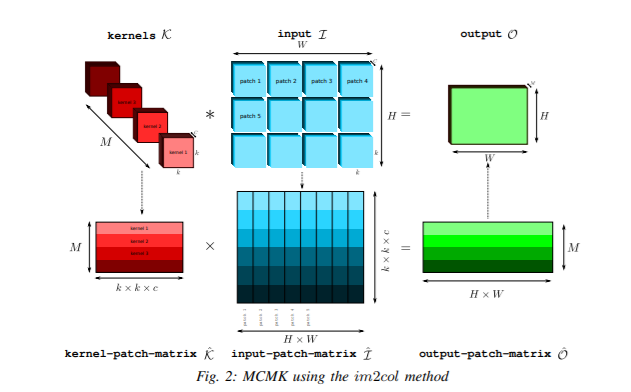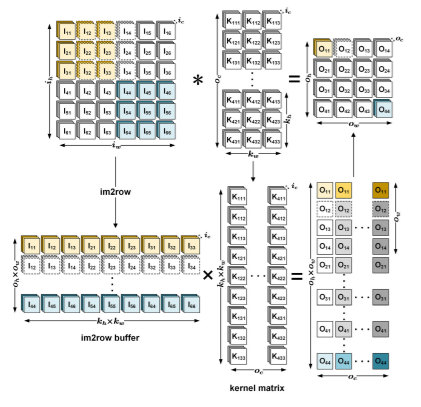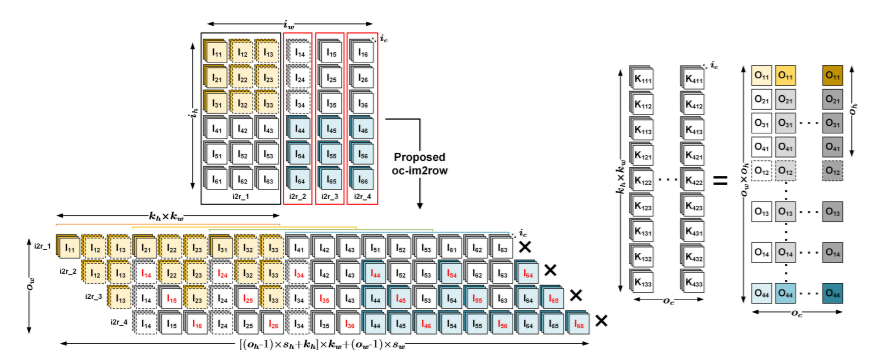×

Search anything:

# Im2row Convolution

#### Machine Learning (ML)Get this book -> Problems on Array: For Interviews and Competitive Programming

In this article, we will understand about Im2row Convolution which is an approach to perform Convolution by modifying the layout of image and filter. It is a better alternative to Im2col.

1. Introduction
2. Im2col Convolution.
2.1 Drawbacks of Im2col Convolution.
3. Im2row Convolution.
3.2 Improvised Im2row Algorithm.

Prerequisite: Im2col Convolution

Before discussing Im2row Convolution, we will also look at im2col and it's drawbacks.

# Introduction:

For a range of significant real-world situations, convolutional neural networks (CNNs) are one of the most successful machine learning algorithms. Both training and inference with CNNs need a significant amount of computing. Convolutional neural networks (CNNs) are made up of networks of typical components such convolutional layers, activation layers, and fully-connected layers. The convolutional layers conduct the majority of the computation in most effective CNNs.

CNNs are cutting-edge models for image classification, object recognition, semantic, instance, and panoptic segmentation, picture super-resolution, and denoising, among other computer vision tasks. CNNs are utilised in speech synthesis and Natural Language Processing in addition to computer vision. Typically, between 50-90% of inference time in CNN models is spent in Convolution.

Now, let’s get back to out topic, Im2row convolution, Im2row Convolution is actually a better version of im2col convolution. So what are the drawbacks of Im2col Convolution, let’s understand that first.

# Im2col Convolution:

Convolutions have become an important feature of current neural networks because of its capacity to collect local information and minimize the number of parameters with weight sharing.

Im2col convolution, also known as Image Block to Column, is a method that involves flattening each window and stacking it as columns in a matrix.

Expanding the image into a column matrix (im2col) and performing Multiple Channel Multiple Kernel (MCMK) convolution using an existing parallel General Matrix Multiplication (GEMM) library is a typical way to constructing convolutional layers. The memory footprint of the input matrix is considerably increased by this im2col conversion, which lowers data locality.

The below figure depicts Multiple Channel Multiple Kernel(MCMK) convolution using im2col.## Now, let’s look at the drawback of im2col:

• Im2col transformations are expensive as well as memory-intensive.
• Removing im2col conversions improves performance by up to 62 percent.
• When im2col accounts for a considerable portion of the Convolution runtime, the speed advantage is especially noticeable for Convolutions with a limited number of output channels. (im2col is not suitable for large number of output channels).
• The space explosion created by creating the column matrix is one of im2col's significant drawbacks.
• Im2col may perform well on GPU, but is a poor choice for CPU implementation.

Now, lets have a look at im2row convolution.

# Im2row Convolution:

Im2row is a method wherein the local patches are unrolled into rows of the input-patch matrix I and the kernels are unrolled into columns of the kernel-patch-matrix K. Im2row works better compared to im2col.

## Let’s look at the traditional im2row based algorithm.The im2row process of I is shown on the left side of Fig. Each convolutional window in the input is flattened to 1D and stored in an im2row buffer row. The im2row buffer then includes input feature blocks in oh x ow rows and kh x kw columns. The relevant output feature block is calculated for each row of the im2row buffer. The conversion of K is shown in the centre of Fig. Each kernel of K is flattened into a column of a kernel matrix with this conversion. Each column of the kernel matrix is utilised to determine the output feature's corresponding channel. As a result, we store the kernel matrix in column-major order for greater spatial locality. However, this algorithm of im2row occupies enormous memory space and needs to copy numerous data.

## So, let’s look at a fast convolution approach that reduces the amount of data transferred by im2row while keeping the buffer size minimal.To acquire contiguous 1 x 9 feature blocks, a 3 x 3 input feature window matching to O-11 is first unfolded in a row-major fashion. Then, as shown in Fig. for i2r_1, the 1 x 9 feature blocks are put at the start of a reusable im2row buffer. The extra 1 x 3 input feature blocks for the computation of O-21 are then unfolded and added to the im2row buffer, resulting in a total of 12 feature blocks. This process is continued until all of the input feature blocks for the first column of O have been unfolded. Finally, the im2row buffer stores 18 contiguous feature blocks. The aforementioned technique is referred to as i2r 1. When i2r_1 is complete, the im2row buffer is indexed with a fixed offset to create an equivalent im2row matrix.

The first column of O is then calculated by matrix multiplying the corresponding im2row matrix with the convolutional kernels, as seen in the first four rows of the output on the right side of Fig. The im2row buffer size and the quantity of data transferred are both identical in i2r_1.

After computing the first column of O, a second im2row unfolding, dubbed i2r_2, is done. It's evident that i2r_2 and i2r_1 share some data. The input features in the second and third columns are shared in the example presented in Fig., but the input features in the fourth column are novel. As a result, as shown in red in Fig. 1, i2r_2 only has to update the data in the fourth column of I into the reusable im2row buffer. After i2r_2, the analogous im2row matrix is matrix multiplied with the convolutional kernels to get the second column of O, same like in i2r_1.

Advantage of the following proposed algorithm:

• The quantity of replicated data in im2row is approximately equal to the size of the input feature map, making it the smallest of the im2row-based convolutions.
• Although the im2row buffer size of the proposed algorithm is larger than that of CMSIS-NN, it is still much smaller than both the MEC and the traditional im2rowbased convolution. Furthermore, in the common case, the size of the im2row buffer is smaller than the input feature map.

## Naive implementation of im2row:

``````def im2row(x, kernel):
kernel_shape = kernel.shape
rows = []

# Assuming Padding = 0, stride = 1
for row in range(x.shape - 1):
for col in range(x.shape - 1):
window = x[row: row + kernel_shape, col: col + kernel_shape]
rows.append(window.flatten())

return np.transpose(np.array(rows))
``````

## Now let's flatten the kernel and do matrix multiplication.

``````output_shape = (input_matrix.shape - kernel.shape) + 1

im2row_matrix = im2row(input_matrix, kernel)

im2row_conv = np.dot(kernel.flatten(), im2row_matrix) + bias
im2row_conv = im2row_conv.reshape(output_shape,output_shape)

print(im2row_conv)
``````

With this article at OpenGenus, you must have the complete idea of Im2row Convolution.

#### Aman Shrivastav

Aman Shrivastav is a Machine Learning Developer, Intern at OpenGenus. He is, currently pursuing B. Tech in Computer Science at Mumbai University.